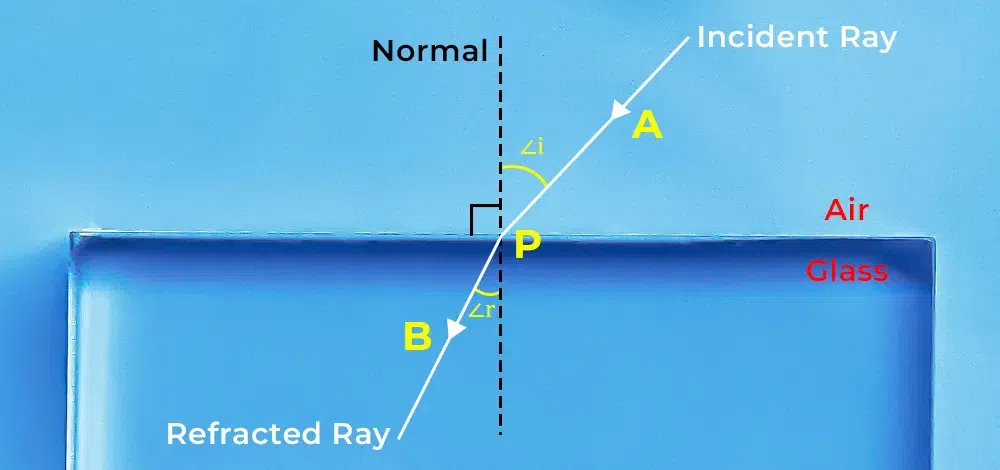GFG App
Open AppBrowser
Continue

Laws of refraction of light are the basic laws which govern the refraction of light. As we know that light changes its path when it changes its medium and this property is called the refraction of light. The laws of refraction of light are useful in defining the relationship between incident light and refracted light.

When we put a pencil in a glass of water, we observe that the pencil is slightly bent but actually it is not. We also observed that during summer, the trees and houses on the other side of an open ground appear to be shaking. While travelling on a road on a hot summer day, we sometimes observed that water appears in the middle of the road out of nowhere and when we get closer, there is no water. The reason behind all this is the “Refraction of light“. In this article, we will discuss the Refraction of light, the Laws of Refraction and its examples in detail.

## What is Refraction of Light?

The phenomenon of change in the direction/path of light rays when it travels from one medium to another is called refraction of light.

The bending of light is caused by the change in the wave’s speed. Light has different speeds in different mediums when light travels in the same medium, it travels along a straight line but when the medium changes the speed of light changes and light bends.

The image below shows the light ray entering the air-glass surface and bending towards the normal. This phenomenon represents the refraction of light.## Laws of Refraction of Light

There are two basic laws of refraction of light which are,

• First Law of Refraction of Light
• Second Law of Refraction of Light

### First Law of Refraction of Light

It states that the incident ray, refracted ray, and normal to the interface at the point of incidence all lie in the same plane.

### Second Law of Refraction of Light

It states that the ratio of the sine of the angle of incidence (i) and the sine of the angle of refraction (r) is constant. It is given as,Where μ is the refractive index of the medium.

### Snell’s Law

The second Law of Refraction of Light is also called Snell’s Law. According to Snell’s Law, for the light if given colour and for the given pair of media, the ratio of the sine of the angle of incidence to the sine of the angle of refraction is constant. It gives the degree of refraction and also tells the relation between the angle of incidence, the angle of refraction and the refractive index of media.where,

• μ is the refractive index of the medium.

## Solved Examples on Laws of Refraction of Light

Example 1: The refractive index of air to water is 5/3. The angle of incidence is sin 50 °. Find the angle of refraction.

Solution:

Given that,

i = 50°, and μ = 5/3

Since,

μ = sin 50°/ sin r

⇒ sin r = sin 50° × (3/5)

⇒ sin r = 0.766 × (3/5)

⇒ sin r = 0.4596

⇒ r = sin-1(0.4596)

⇒ r = 27.36°

Therefore, the angle of refraction will be 27.36°.

Example 2: The velocity of light in glass is 2 × 108m/s and the velocity of light in air is 3 × 108m/s. Find the refractive index of glass.

Solution:

Given that,

v = 2 × 108 m/s

c = 3 × 108 m/s

Since,

μ = c/v

Therefore,

μ = 3 × 108/ 2 ×108

⇒ μ  = 1.5

Therefore, refractive index will be 1.5.

Example 3: Light travelling through an optical fibre (n=1.45) incident at the end of the fibre at 30°. Determine the angle of refraction beyond the fibre.

Solution:

Given that,

Refractive Index of the Fiber, μ is 1.45

Angle of Incidence, ∠i is 30°.

Since,

μ1 × sin i = μ2 × sin r

⇒ 1.45 × sin 30° = 1 × sin r

⇒ 1.45 × 1/2 = sin r

⇒ r = sin-1(0.725)

⇒ r = 46.46°

Example 4: Consider a ray is refracted at an angle of 20° and the refractive index is 1.5. Determine the angle of incidence by the ray.

Solution:

Given that,

Refractive Index, μ is 1.5

Angle of Refraction, ∠r is 20°.

Since,

μ = sin i / sin r

⇒ 1.5 = sin i / sin 20°

⇒ sin i = 0.513

⇒ i = sin-1(0.513)

⇒ i = 30.86°

## FAQs on Laws of Refraction of Light

### Q1: What is the Refraction of Light?

Refraction of Light is the phenomenon which allows light to bend while travelling from one medium to another.

### Q2: What are the 2 laws of refraction?

The two laws of refraction are,

First Law: The incident ray, refracted ray, and normal to the interface at the point of incidence all lie in the same plane.

Second Law: The ratio of the sine of the angle of incidence (i) and the sine of the angle of refraction (r) is constant.

### Q3: How many laws of refraction are there?

There are two Laws of Refraction,

• First Law of Refraction
• Second Law of Refraction

### Q4: What is the difference between Refraction and Reflection of light?

Reflection: The bouncing back of light from a smooth and shining surface is called reflection. Angle of incidence is equal to the angle of reflection. ∠i = ∠r.

Refraction: The bending of light rays, when it travels from one medium to another is called refraction of light. The angle of incidence and the refracted ray may or may not be equal.